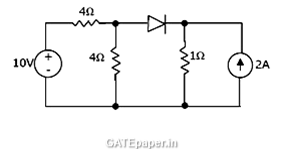### GATE 1997 Video Solution on EDC (Electronic Devices and Circuits)

1. For a MOS capacitor fabricated on a P-type semiconductor, strong inversion occurs when
a. Surface potential is equal to Fermi level
b. Surface potential is zero
c. Surface potential is negative is negative and equal to Fermi potential in magnitude
d. Surface potential is positive and equal to twice the Fermi potential

2. In a common emitter BJT amplifier, the maximum usable supply voltage is limited by
a. Avalanche breakdown of base emitter junction
b. Collector base breakdown voltage with emitter open (BVCBO)
c. Collector emitter breakdown voltage with base open (BVCEO)
d. Zener breakdown voltage of the emitter base junction

3. In the circuit shown, the current flowing through the ideal diode equal toa. 0 Amp
b. 4 Amp
c. 1 Amp
d. None of the above

4. The intrinsic carrier density at 300oK is 1.5 X 1010 per cm3 for silicon. For n-type silicon doped to 2.25 X 1015 atoms/cm3, the equilibrium electron and hole densities are
a. n = 1.5 x 1015/cm3, p = 1.5 x 1010 /cm3
b. n = 1.5 x 1010/cm3, p = 2.25 x 1015 /cm3
c. n = 2.25 x 1015/cm3, p = 1.5 x 105 /cm3
d. n = 1.5 x 1010/cm3, p = 1.5 x 1010 /cm3

5. The gate delay of an NMOS inverter is dominated by charge time rather than discharge time because
a. The driver transistor has larger threshold voltage than the load transistor
b. The driver transistor has larger leakage currents compared to the load transistor
c. The load transistor has a smaller W/L ratio compared to the driver transistor
d. None of the above

6. In the cascade amplifier circuit shown, determine the values of R1, R2 and RL such that the quiescent current through the transistors is 1 mA and the collector voltages are VC1 = 3 volts, and VC2 = 6 volts. Take VBE = 0.7 volts and assume β of the transistors is very high and base currents to be negligible.Answer: 59 kΩ, 31 kΩ, 3 kΩ

7. Given NMOS circuit as shown. The specifications of the circuit are :
VDD = 10 volts, β = µnCox(W/L) = 10-4 Amp/V2, VT = 1 volt and IDS = 0.5 mA.
Evaluate VDS and RD. Neglect body effect.8. Find static noise margins for a BJT inverter shown in figure. Transistor used is an NPN type with the specifications as follows.Answer: (NM)L = 1.3 volts, (NM)H = 3.5 volts

9. For a typical NPN transistor, the following data are available :
WC = 20 µm and collector doping = 5 X 1018 cm-3
WE = 1 µm and emitter doping = 10 X 1019 cm-3
Base doping = 5 X 1015 cm-3
Minority carrier life time in the base region is τn = 5 µsec.Answer: τt = WB2/2DB, β = τnt and α = β/β+1

10. An n-type silicon bar is doped uniformly by phosphorous atoms to a concentration 4.5 X 1013 cm-3. The bar has cross section of 1 mm2 and length of 10 cm. It is illuminated uniformly for region x < 0 as shown.
Assume optical generation rate as 1021 electron-hole pairs per cm3 per second, the hole lifetime and electron lifetime are equal to 1 µsec.
Evaluate the hole and electron diffusion currents at x = 36.4 µm.